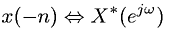Equations > Signal Processing > Fourier Transform Properties > Discrete-Time Fourier time reversal property; x(n) real

### Discrete-Time Fourier time reversal property; x(n) realLatex Code:

MathML Code:

 $x\left(-n\right)⇔{X}^{*}\left({e}^{j\omega }\right)$

MathType 5.0: